Q-Chem 5.0 User’s Manual

# A.5 Constrained Optimization

Constrained optimization refers to the optimization of molecular structures in which certain parameters (e.g., bond lengths, bond angles or dihedral angles) are fixed. In quantum chemistry calculations, this has traditionally been accomplished using Z-matrix coordinates, with the desired parameter set in the Z-matrix and simply omitted from the optimization space. In 1992, Baker presented an algorithm for constrained optimization directly in Cartesian coordinates . Baker’s algorithm used both penalty functions and the classical method of Lagrange multipliers , and was developed in order to impose constraints on a molecule obtained from a graphical model builder as a set of Cartesian coordinates. Some improvements widening the range of constraints that could be handled were made in 1993 . Q-Chem includes the latest version of this algorithm, which has been modified to handle constraints directly in delocalized internal coordinates .

The essential problem in constrained optimization is to minimize a function of, for example,variables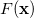subject to a series of m constraints of the form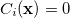,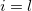.. Assuming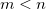, then perhaps the best way to proceed (if this were possible in practice) would be to use theconstraint equations to eliminateof the variables, and then solve the resulting unconstrained problem in terms of the (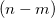independent variables. This is exactly what occurs in a Z-matrix optimization. Such an approach cannot be used in Cartesian coordinates as standard distance and angle constraints are non-linear functions of the appropriate coordinates. For example a distance constraint (between atomsand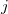in a molecule) is given in Cartesians by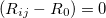, with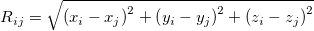(A.17)

and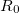the constrained distance. This obviously cannot be satisfied by elimination. What can be eliminated in Cartesians are the individual,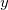andcoordinates themselves and in this way individual atoms can be totally or partially frozen.

Internal constraints can be handled in Cartesian coordinates by introducing the Lagrangian function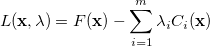(A.18)

which replaces the functionin the unconstrained case. Here, the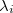are the so-called Lagrange multipliers, one for each constraint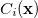. Differentiating Eq. (A.18) with respect to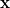and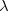affords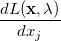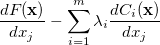(A.19)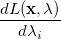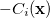(A.20)

At a stationary point of the Lagrangian we have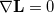, i.e., all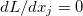and all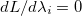. This latter condition means that alland thus all constraints are satisfied. Hence, finding a set of values (,) for whichwill give a possible solution to the constrained optimization problem in exactly the same way as finding anfor which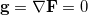gives a solution to the corresponding unconstrained problem.

The Lagrangian second derivative matrix, which is the analogue of the Hessian matrix in an unconstrained optimization, is given by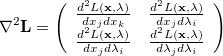(A.21)

where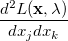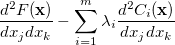(A.22)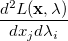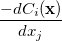(A.23)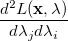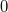(A.24)

Thus, in addition to the standard gradient vector and Hessian matrix for the unconstrained function, we need both the first and second derivatives (with respect to coordinate displacement) of the constraint functions. Once these quantities are available, the corresponding Lagrangian gradient, given by Eq. (A.19), and Lagrangian second derivative matrix, given by Eq. (A.21), can be formed, and the optimization step calculated in a similar manner to that for a standard unconstrained optimization .

In the Lagrange multiplier method, the unknown multipliers,, are an integral part of the parameter set. This means that the optimization space consists of allvariablesplus allLagrange multipliers, one for each constraint. The total dimension of the constrained optimization problem,, has thus increased bycompared to the corresponding unconstrained case. The Lagrangian Hessian matrix,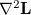, hasextra modes compared to the standard (unconstrained) Hessian matrix,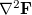. What normally happens is that these additional modes are dominated by the constraints (i.e., their largest components correspond to the constraint Lagrange multipliers) and they have negative curvature (a negative Hessian eigenvalue). This is perhaps not surprising when one realizes that any motion in the parameter space that breaks the constraints is likely to lower the energy.

Compared to a standard unconstrained minimization, where a stationary point is sought at which the Hessian matrix has all positive eigenvalues, in the constrained problem we are looking for a stationary point of the Lagrangian function at which the Lagrangian Hessian matrix has as many negative eigenvalues as there are constraints (i.e., we are looking for anth-order saddle point). For further details and practical applications of constrained optimization using Lagrange multipliers in Cartesian coordinates, see .

Eigenvector following can be implemented in a constrained optimization in a similar way to the unconstrained case. Considering a constrained minimization withconstraints, then Eq. (A.11) is replaced by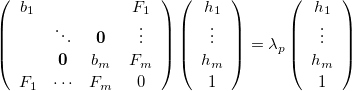(A.25)

and Eq. (A.12) by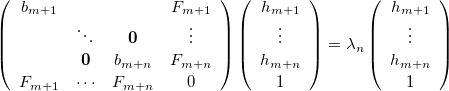(A.26)

where now the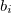are the eigenvalues of, with corresponding eigenvectors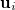, and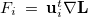. Here Eq. (A.25) includes theconstraint modes along which a negative Lagrangian Hessian eigenvalue is required, and Eq. (A.26) includes all the other modes.

Equations (A.25) and (A.26) implement eigenvector following for a constrained minimization. Constrained transition state searches can be carried out by selecting one extra mode to be maximized in addition to theconstraint modes, i.e., by searching for a saddle point of the Lagrangian function of order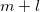.

It should be realized that, in the Lagrange multiplier method, the desired constraints are only satisfied at convergence, and not necessarily at intermediate geometries. The Lagrange multipliers are part of the optimization space; they vary just as any other geometrical parameter and, consequently the degree to which the constraints are satisfied changes from cycle to cycle, approaching 100% satisfied near convergence. One advantage this brings is that, unlike in a standard Z-matrix approach, constraints do not have to be satisfied in the starting geometry.

Imposed constraints can normally be satisfied to very high accuracy,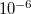or better. However, problems can arise for both bond and dihedral angle constraints near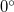and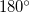and, instead of attempting to impose a single constraint, it is better to split angle constraints near these limiting values into two by using a dummy atom Baker:1993b, exactly analogous to splitting abond angle into two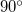angles in a Z-matrix.

Note: Exactandsingle angle constraints cannot be imposed, as the corresponding constraint normals,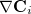, are zero, and would result in rows and columns of zeros in the Lagrangian Hessian matrix.